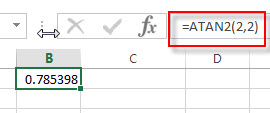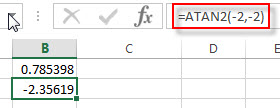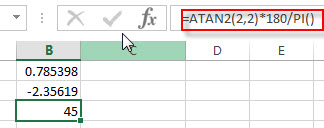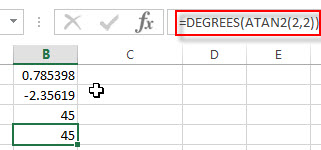## Excel ATAN2 Function

This post will guide you how to use Excel ATAN2 function with syntax and examples in Microsoft excel.

### Description

The Excel ATAN2 function returns the arctangent, or inverse tangent, of the specified x- and y-coordinates. And you can use the ATAN2 function to calculate the arctangent of a given x and y coordinates. The returned value is an angle between –pi/2 and pi/2.

The ATAN2 function is a build-in function in Microsoft Excel and it is categorized as a Math and Trigonometry Function.

The ATAN2 function is available in Excel 2016, Excel 2013, Excel 2010, Excel 2007, Excel 2003, Excel XP, Excel 2000, Excel 2011 for Mac.

### Syntax

The syntax of the ATAN2 function is as below:

`= ATAN2 (x_num, y_num)`

Where the ATAN2 function arguments are:

• x_num -This is a required argument. The x-coordinate of a point.
• Y_num – This is a required argument. The y-coordinate of a point.

Note:

• If the values of both x_num and y_num arguments are 0, the ATAN2 will return the #DIV/0! Error.
• The returned value of ATAN2(a,b) is equal to the formula ATAN2(b,a).
• If you want to express the arctangent in degrees, you just need to multiply the result by 180/PI() or you can use the DEGREES function.
• If the supplied number is not a numeric value, the ATAN function will get the #VALUE！ Error。
• If the returned result is a positive value, it indicates that it is a counterclockwise angle from the x-axis. If the returned result is a negative value, it indicates that it is a clockwise angle.

### Excel ATAN2Function Examples

The below examples will show you how to use Excel ATAN2 Function to return the arctangent of x and y coordinates.

1# get the arctangent of the x- and y-coordinates 2,2 in radians, enter the following formula in Cell B1.

`=ATAN2(2,2)`The returned result is 0.785398.

2# get the arctangent of the x- and y-coordinates -2,-2 in radians, -3*pi/4, using the following formula in Cell B2.

`=ATAN2(-2,-2)`This formula will return the value -2.35619.

3# get the arctangent of the x- and y-coordinates 2,2 in degrees, using the following formula in Cell B3.

`=ATAN2(2,2)*180/PI()`Or you can use the below formula to achieve the same result:

`=DEGREES(ATAN2(2.2))`### Related Functions

• Excel ATAN function
The Excel ATAN function returns the arctangent of a number. So this function can be used to calculates the arctangent of a supplied number. The returned angle is given in radians in the range –pi/2 to pi/2. The syntax of the ATAN function is as below:= ATAN (number)…
• Excel ATANH function
The Excel ATANH function returns the inverse hyperbolic tangent of a given number. The syntax of the ATANH function is as below:= ATANH (number)…
• Excel ACOS function
The Excel ACOS function returns the arccosine value of a number.The ACOS function is a build-in function in Microsoft Excel and it is categorized as a Math and Trigonometry Function.The syntax of the ACOS function is as below:= ACOS(number)…
• Excel ACOSH function
The Excel ACOSH function returns the inverse hyperbolic cosine of a number.The syntax of the ACOSH function is as below:= ACOSH (number)…
• Excel ASIN function
The Excel ASIN function returns the arcsine value of a number.The syntax of the ASIN function is as below:= ASIN (number)…
• Excel DEGREES Function
The Excel DEGREES function converts radians into degrees. And it will return a numeric value. The syntax of the DEGREES function is as below:=DEGREES (angle)…